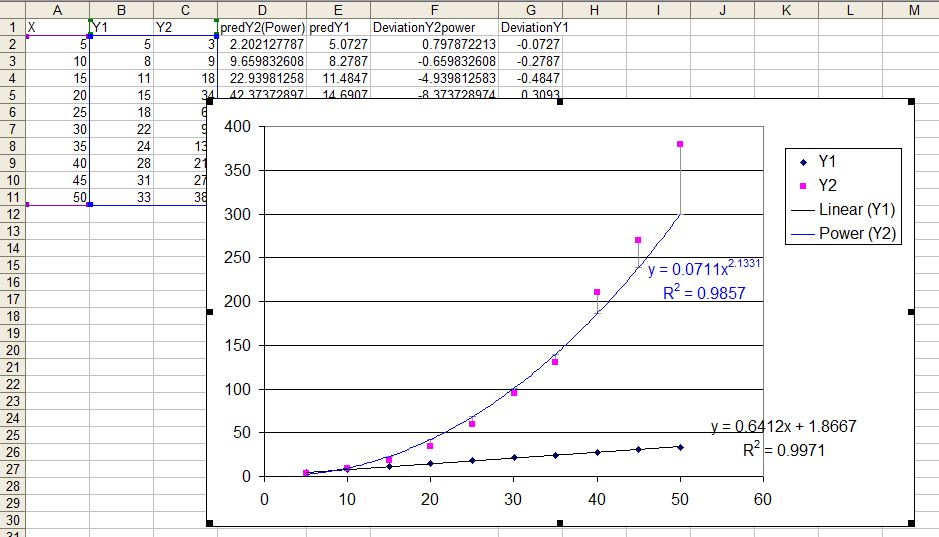## Curve fitting:

(1) Create a scatter plot and select series and select add trend line(2) If you like to see R square and equation on the plot, check the boxes. You can also set intercept. You can repeat this for each series and also more than one curve for each series

(3) You can plot deviations (errors for each model - i.e. difference between predicted and observed) as shown in above chart.
For this you need to create a new column for predicted values using a formula based on the equation displayed:

for example:
predY2(Power)  =(0.0711*A2^2.1331)

predY1 =(0.6412*A2+1.8667)

Then calculate the difference between Y2 (observed and predicted):
DeviationY2power = (C2-D2)
DeviationY1 = (B2-E2)

Now we want to add error bars and add to minus side only and select the difference range.(4) Beside the provided equations, we can also fit own equation. For example if we are interested to plot two equations:
y = 55 + x0
y = 7.8x-95

Now we need to create a new series calculating the expected observations for each level of X.
YpredE1 = 55+A2*0
YpredE2 = (7.8*A2-95)

Now add new data to the plot, go to source data, add new series. Then select the series and than again go back to chart type and select smooth line.